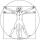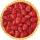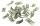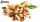Equation

Solve the equation: 1/2-2/8 = 1/10; Write the result as a decimal number.

Result

x =  0.4

Solution:

1/2x-2/8x=1/10

10x = 4

x = 25 = 0.4

Calculated by our simple equation calculator.

Leave us a comment of this math problem and its solution (i.e. if it is still somewhat unclear...):Be the first to comment!To solve this verbal math problem are needed these knowledge from mathematics:

Do you have a linear equation or system of equations and looking for its solution? Or do you have quadratic equation?

Next similar math problems:

1. Simple equationSolve for x: 3(x + 2) = x - 18
2. Equation with xSolve the following equation: 2x- (8x + 1) - (x + 2) / 5 = 9
3. Proportionwrite the following proportion 8 is to 64 as to 2 is to x
4. Unknown numberIdentify unknown number which 1/5 is 40 greater than one tenth of that number.
5. One-thirdA one-third of unknown number is equal to five times as great as the difference of the same unknown number and number 28. Determine the unknown number.
6. FractionsThree-quarters of an unknown number are 4/5. What is 5/6 of this unknown number?
7. Strange xFor what x is true ??
8. FactoryIn the factory workers work in three shifts. In the first inning operates half of all employees in the second inning and a third in the third inning 200 employees. How many employees work at the factory?
9. GoodsTo the market is introducing a new product, the first week is sold at a reduced price. 8 products is sell at an entry price same as 5 products at the normal price. How much % is reduced price less than the current price for this product?
10. Spain vs USASpain lost to the US by 4 goals. In the match total fell 10 goals. How many goals gave the Spain and how the United States?
11. Bag of peanutsJoe eat 1/3 of a bag of peanuts, mark eat 1/4 of the remaining in the bag of peanuts, Alvin eat 1/2 of the remaining bag of peanuts, peter eat 10 peanuts, there are 71 peanuts left. Hon many peanuts were in the bags?
12. Cleaning windowsCleaning company has to wash all the windows of the school. The first day washes one-sixth of the windows of the school, the next day three more windows than the first day and the remaining 18 windows washes on the third day. Calculate how many windows ha
13. CandyPeter had a sachet of candy. He wanted to share with his friends. If he gave them 30 candies, he would have 62 candies. If he gave them 40 candies, he would miss 8 candies. How many friends did Peter have?
14. GardenThe garden around the new majer is divided as follows: 35% vegetable, 30% fruit orchards, 10% flowers and the remaining 120 m2 are lawns. What is the total area of the garden?
15. Equation?
16. 1.5 divided1.5 divided by 1 = w divided by 4
17. Two trainsThere were 159 freight wagons on the railway station creating 2 trains. One had 15 more wagons than the other. How many wagons did each train have?